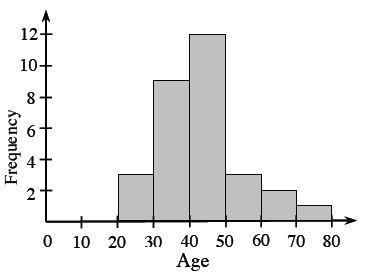### Home > ACC7 > Chapter 7 Unit 4 > Lesson CC2: 7.1.6 > Problem7-75

7-75.

Ages of golfers participating in a golf tournament were $44$, $48$, $40$, $25$, $28$, $37$, $29$, $34$, $45$, $51$, $43$, $35$, $38$, $57$, $50$, $35$, $47$, $30$, $61$, $43$, $44$, $60$, $46$, $43$, $33$, $45$, $42$, $34$, $32$, and $74$.

1. Create a stem-and-leaf plot for this data.

Sort the data from low to high.

The “stem” part of the graph represents all of the digits in a number except the last one. The “leaf” part of the graph represents the last digit of each of the numbers.

 $2$ $5$  $8$  $9$ $3$ $0$  $2$  $3$  $4$  $4$  $5$  $5$  $7$  $8$ $4$ $0$  $2$  $3$  $3$  $3$  $4$  $4$  $5$  $5$  $6$  $7$  $8$ $5$ $0$  $1$  $7$ $6$ $0$  $1$ $7$ $4$
2. Use the stem-and-leaf plot to create a histogram.

In histograms, the intervals for the data are shown on the horizontal axis and the frequency (number of pieces of data in each interval) is represented by the height of a bar above the interval.3. Describe the shape and spread of the data. Are there any apparent outliers?

Is the graph symmetric? How many peaks does it have?
Are any of the data points significantly large or small compared to the rest of the data?

4. Use the appropriate measure of center to describe the “typical” age of golfers at the tournament.

What is the value of the median? What is the value of the mean? Considering the outlier, which
value do you think is better suited to describe the typical age of the golfers at the tournament?